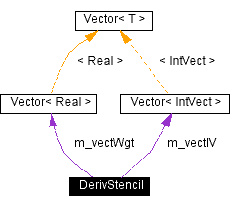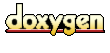Main Page   Namespace List   Class Hierarchy   Alphabetical List   Compound List   File List   Compound Members   File Members

# DerivStencil Class Reference

class to encapsulate the operations to create derivs on irreg stencils More...

`#include <DerivStencil.H>`

Collaboration diagram for DerivStencil:[legend]
List of all members.

## Public Methods

void define ()
bool isDefined () const
DerivStencil ()
DerivStencil (const DerivStencil &a_dsin)
~DerivStencil ()
void clear ()
int size () const
const IntVectgetIndex (int a_ivec) const
const RealgetWeight (int a_ivec) const
void accumulate (const IntVect &a_iv, Real a_weight)
const DerivStencil & operator= (const DerivStencil &a_dsin)
const DerivStencil & operator *= (Real a_facin)
const DerivStencil & operator/= (Real a_denom)
const DerivStencil & operator+= (Real a_facin)
const DerivStencil & operator-= (Real a_facin)

## Protected Attributes

Vector< IntVectm_vectIV
Vector< Realm_vectWgt
bool isdefined

## Detailed Description

class to encapsulate the operations to create derivs on irreg stencils

DerivStencil is meant to be used to encapsulate the information necessary to take finite difference derivatives at a point in space. Every point in the stencil has a weight. You add them (the boxarrayindex and the weight) at the same time and you can manipulate the weights enmasse by real number operations. Stencils may not interact with each other with the same sort of arithmetic because that would bring up issues as to what to do when there is incomplete intersection between the stencils.

## Constructor & Destructor Documentation

 DerivStencil::DerivStencil ( )
 default constructor; creates empty vectors

 DerivStencil::DerivStencil ( const DerivStencil & a_dsin )
 copy constructor; sets *this = a_dsin

 DerivStencil::~DerivStencil ( )

## Member Function Documentation

 void DerivStencil::accumulate ( const IntVect & a_iv, Real a_weight )
 add another set if the IntVect is not in the stencil already. \ Add the weight to the existing weight otherwise**

 void DerivStencil::clear ( )
 make derivstencil empty

 void DerivStencil::define ( )

 const IntVect& DerivStencil::getIndex ( int a_ivec ) const
 get iv at ivec

 const Real& DerivStencil::getWeight ( int a_ivec ) const
 get weight at ivec

 bool DerivStencil::isDefined ( ) const` [inline]`
 return true if any define function been called.

 const DerivStencil& DerivStencil::operator *= ( Real a_facin )
 Multiply each weight by a_facin does nothing if vectors are of zero length

 const DerivStencil& DerivStencil::operator+= ( Real a_facin )
 add a_facin to each weight does nothing if vectors are of zero length

 const DerivStencil& DerivStencil::operator-= ( Real a_facin )
 subtract a_facin from each weight does nothing if vectors are of zero length

 const DerivStencil& DerivStencil::operator/= ( Real a_denom )
 divide each weight by a_denom does nothing if vectors are of zero length. Be advised --- This function does no checking to see if a_denom is close to zero.

 const DerivStencil& DerivStencil::operator= ( const DerivStencil & a_dsin )
 assignment operator

 int DerivStencil::size ( ) const
 return length of vectors

## Member Data Documentation

 bool DerivStencil::isdefined` [protected]`

 Vector DerivStencil::m_vectIV` [protected]`

 Vector DerivStencil::m_vectWgt` [protected]`

The documentation for this class was generated from the following file:
Generated on Thu Aug 29 11:07:40 2002 for Chombo&INS by1.2.16